Courses

# Test: Multiplication - 1

## 10 Questions MCQ Test Mathematics for Class 3: NCERT | Test: Multiplication - 1

Description
This mock test of Test: Multiplication - 1 for Class 3 helps you for every Class 3 entrance exam. This contains 10 Multiple Choice Questions for Class 3 Test: Multiplication - 1 (mcq) to study with solutions a complete question bank. The solved questions answers in this Test: Multiplication - 1 quiz give you a good mix of easy questions and tough questions. Class 3 students definitely take this Test: Multiplication - 1 exercise for a better result in the exam. You can find other Test: Multiplication - 1 extra questions, long questions & short questions for Class 3 on EduRev as well by searching above.
QUESTION: 1

### Each of these packs has exactly 5 buttons in it. How many buttons are there in all?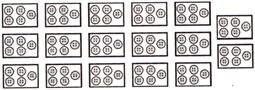Solution:

Total number of packs = 17
Number of buttons in one pack = 5
Total number of buttons in all 17 packs = 17 x 5 = 85

QUESTION: 2

### Raj a bought these pencils. Each pencil cost Rs.2. How much did the pencils cost all together?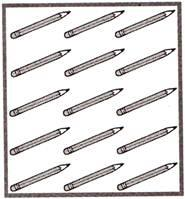Solution:

Cost of each pencil = Rs.2
Total number of pencils = 15
Cost of all 15 pencils = 15 x 2 = Rs. 30

QUESTION: 3

### The word problem below is part of Madhavi's homework. Nani bought 25 packages of balloons. Each package has 4 balloons. How many balloons did she buy in all? Q. Which of the following number sentences can Madhavi use to solve this problem?

Solution:

Total number of packages of balloon = 25
Number of balloons in each package = 4
So, Total number of balloons Nani bought = 25 x 4 = 100

QUESTION: 4

At the craft fair, Gopal sold toy trains like the one shown below.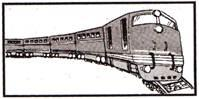He sold each train for Rs. 28. How much did Gopal make by selling 4 trains?

Solution:

Price for selling each toy train = Rs.28
Number of trains Gopal sold = 4
Total money made by Gopall = Rs.28 x 4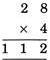QUESTION: 5

7 x 9 = ______

Solution:

7 x 9 = 63

QUESTION: 6

Swaraj wants to buy 3 bags of potato chips. Each bag of potato chips costs Rs. 50. If she uses a coupon for 'Rs. 20 off, how much will Swaraj owe for the 3 bags of potato chips?

Solution:

Total cost of 3 bags of potato chips = Rs. 50 x 3 = Rs. 150

But she uses a discount coupon of Rs. 20

So, Swaraj has to pay Rs. 150 - Rs. 20 = Rs. 130 only

QUESTION: 7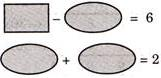If both equations shown above are true, which of the following equations must also be true?

Solution: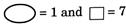So, it satisfies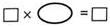Since 7 x 1 = 7

QUESTION: 8

Madhu arranged one rupee coins in the pattern shown below: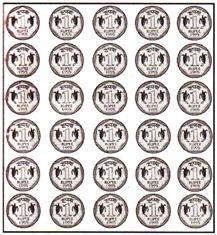What operation best shows how he arranged?

Solution:

Number of coins in one row = 5

Number of coins in one column = 6

Total number of coins in the whole pattern = 6 x 5 = 5 x 6

So, 6 x 5 or 5 x 6 shows the arrangement pattern.

QUESTION: 9

Guru's family eats 4 to 6 apples every day. Which is a reasonable number of days that it will take Guru's family to eat a bag of 20 apples?

Solution:

Between 3 and 6 days.

If the family eats 4 apples per day, then it will take 5 days to eat 20 apples.

If thay eat 6 apples per day, then it will take more than 3 days to eat 20 apples.

QUESTION: 10

Madhuri has 2 pizzas that are cut into 6 slices each. She and 3 friends eat 9 slices. Which of these shows one way to find the number of slices Madhuri has left?

Solution:

Total number of pizza = 2

Number of slices in each pizza = 6

Total number of slices in both pizza = 2 x 6

Madhuri and 3 friends ate 9 slices

So, number of slices left by Madhuri are:
Multiply 2 by 6 and then subtract 9.

Track your progress, build streaks, highlight & save important lessons and more!

### Similar Content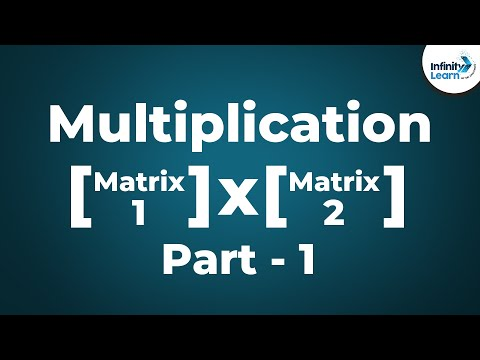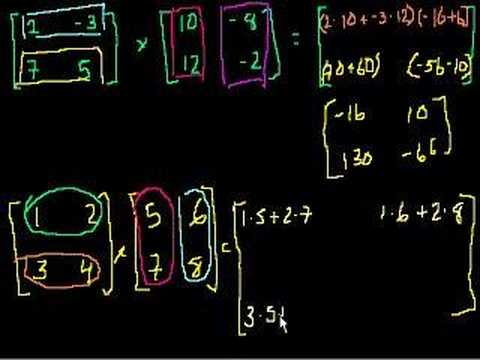### Related tests